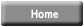Index of refraction of liquids
versus amount of additive

 Introduction: One indicator of the optical density of a material is the index of refraction value of the material. Index of refraction values (represented by the symbol n) are numerical index values which are expressed relative to the speed of light in a vacuum. The index of refraction value of a material is a number which indicates the number of times that a light wave would be in that material rather than in a vacuum. A vacuum is given an n value of 1.0000.We can calculate the index of refraction using the angle that light bends when traveling from one material to another material. If the index of refraction of a solution changes based on the amount of solutes we can use such property to find out the density of the solute without even touching it. For example, sugar factories will be able to know the percent of sugar in a solution while it is passing through a clear pipe.

Project description:
In this project you try to use refraction to determine the concentration of salt or sugar in water. You may use a refractometer for your experiments or build a refractometer as described in project details.
Details of this project
More details or support for this project is available for the members of ScienceProject.com web site. Material needed for experiment or a science kit about this title may be available at MiniScience.com.